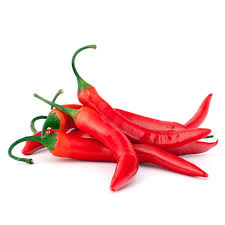# I am a chilli

Probability Level 3You have $\color{#D61F06}{\textbf{20 distinct red chilies}}$ and $\color{#20A900}{\textbf{10 distinct Green chilies}}$.

The number of $\color{#D61F06}{\text{special red chili powders}}$ you can form is $\color{#D61F06}{R}$.

The number of $\color{#20A900}{\text{special green chili powders}}$ you can form is $\color{#20A900}{G}$.

Then the ratio $\dfrac{\color{#D61F06}{R}}{\color{#20A900}{G}}$ is equal to $\dfrac{a}{b}$ where $a$ and $b$ are coprime, positive integers, find $a+b$.

Details and assumptions -

For making a special chili powder, you can at once use exactly $2$ chilies, and of same color.

$\color{#D61F06}{\text{Red chili}}+ \color{#20A900}{\text{Green chili}} \neq$ any of the asked powders.

$\bullet$ This problem is a part of the set Vegetable combinatorics

×